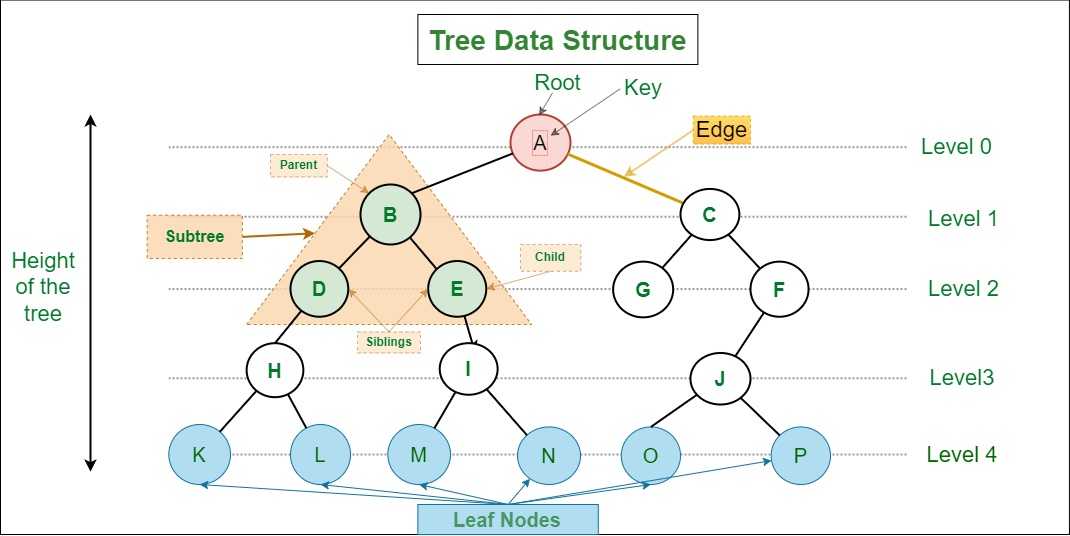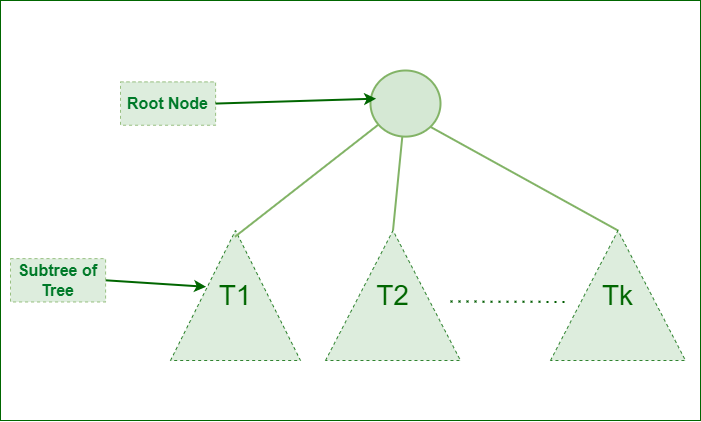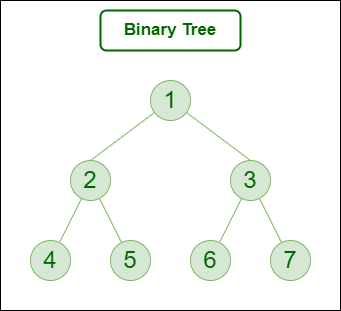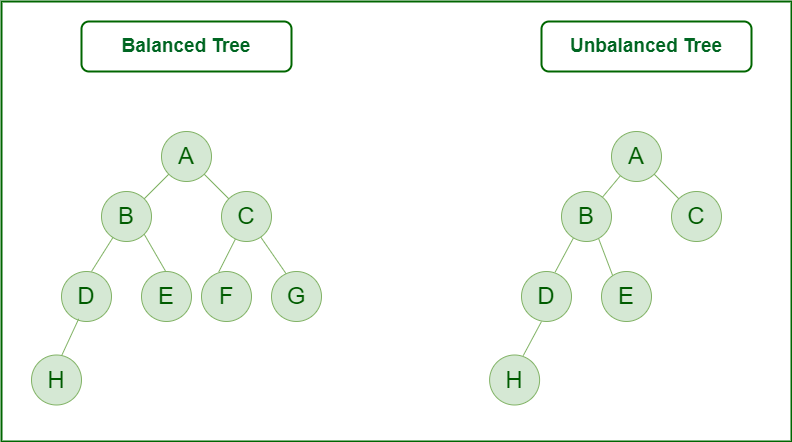Open in App
Not now

# Introduction to Tree – Data Structure and Algorithm Tutorials

• Difficulty Level : Easy
• Last Updated : 27 Jan, 2023

### What is a Tree data structure?

A tree data structure is a hierarchical structure that is used to represent and organize data in a way that is easy to navigate and search. It is a collection of nodes that are connected by edges and has a hierarchical relationship between the nodes. The topmost node of the tree is called the root, and the nodes below it are called the child nodes. Each node can have multiple child nodes, and these child nodes can also have their own child nodes, forming a recursive structure.

This data structure is a specialized method to organize and store data in the computer to be used more effectively. It consists of a central node, structural nodes, and sub-nodes, which are connected via edges. We can also say that tree data structure has roots, branches, and leaves connected with one another.Introduction to Tree – Data Structure and Algorithm Tutorials

### Recursive Definition:

A tree consists of a root, and zero or more subtrees T1, T2, … , Tk such that there is an edge from the root of the tree to the root of each subtree.### Why Tree is considered a non-linear data structure?

The data in a tree are not stored in a sequential manner i.e, they are not stored linearly. Instead, they are arranged on multiple levels or we can say it is a hierarchical structure. For this reason, the tree is considered to be a non-linear data structure.

### Basic Terminologies In Tree Data Structure:

• Parent Node: The node which is a predecessor of a node is called the parent node of that node. {B} is the parent node of {D, E}.
• Child Node: The node which is the immediate successor of a node is called the child node of that node. Examples: {D, E} are the child nodes of {B}.
• Root Node: The topmost node of a tree or the node which does not have any parent node is called the root node. {A} is the root node of the tree. A non-empty tree must contain exactly one root node and exactly one path from the root to all other nodes of the tree.
• Leaf Node or External Node: The nodes which do not have any child nodes are called leaf nodes. {K, L, M, N, O, P} are the leaf nodes of the tree.
• Ancestor of a Node: Any predecessor nodes on the path of the root to that node are called Ancestors of that node. {A,B} are the ancestor nodes of the node {E}
• Descendant: Any successor node on the path from the leaf node to that node. {E,I} are the descendants of the node {B}.
• Sibling: Children of the same parent node are called siblings. {D,E} are called siblings.
• Level of a node: The count of edges on the path from the root node to that node. The root node has level 0.
• Internal node: A node with at least one child is called Internal Node.
• Neighbour of a Node: Parent or child nodes of that node are called neighbors of that node.
• Subtree: Any node of the tree along with its descendant.

### Properties of a Tree:

• Number of edges: An edge can be defined as the connection between two nodes. If a tree has N nodes then it will have (N-1) edges. There is only one path from each node to any other node of the tree.
• Depth of a node: The depth of a node is defined as the length of the path from the root to that node. Each edge adds 1 unit of length to the path. So, it can also be defined as the number of edges in the path from the root of the tree to the node.
• Height of a node: The height of a node can be defined as the length of the longest path from the node to a leaf node of the tree.
• Height of the Tree: The height of a tree is the length of the longest path from the root of the tree to a leaf node of the tree.
• Degree of a Node: The total count of subtrees attached to that node is called the degree of the node. The degree of a leaf node must be 0. The degree of a tree is the maximum degree of a node among all the nodes in the tree.

Some more properties are:

• Traversing in a tree is done by depth first search and breadth first search algorithm.
• It has no loop and no circuit
• It has no self-loop
• Its hierarchical model.

### Syntax:

struct Node
{
int data;
struct Node *left_child;
struct Node *right_child;
};

### Basic Operation Of Tree:

Create – create a tree in data structure.
Insert − Inserts data in a tree.
Search − Searches specific data  in a tree to check it is present or not.
Preorder Traversal – perform Traveling a tree in a pre-order manner in data structure .
In order Traversal – perform Traveling a tree in an in-order manner.
Post order Traversal –perform Traveling a tree in a post-order manner.

### Example of Tree data structureHere,

Node 1 is the root node

1 is the parent of 2 and 3

2 and 3 are the siblings

4, 5, 6 and 7 are the leaf nodes

1 and 2 are the ancestors of 5

## C++

 `// C++ program to demonstrate some of the above``// terminologies``#include ``using` `namespace` `std;``// Function to add an edge between vertices x and y``void` `addEdge(``int` `x, ``int` `y, vector >& adj)``{``    ``adj[x].push_back(y);``    ``adj[y].push_back(x);``}``// Function to print the parent of each node``void` `printParents(``int` `node, vector >& adj,``                  ``int` `parent)``{``    ``// current node is Root, thus, has no parent``    ``if` `(parent == 0)``        ``cout << node << ``"->Root"` `<< endl;``    ``else``        ``cout << node << ``"->"` `<< parent << endl;``    ``// Using DFS``    ``for` `(``auto` `cur : adj[node])``        ``if` `(cur != parent)``            ``printParents(cur, adj, node);``}``// Function to print the children of each node``void` `printChildren(``int` `Root, vector >& adj)``{``    ``// Queue for the BFS``    ``queue<``int``> q;``    ``// pushing the root``    ``q.push(Root);``    ``// visit array to keep track of nodes that have been``    ``// visited``    ``int` `vis[adj.size()] = { 0 };``    ``// BFS``    ``while` `(!q.empty()) {``        ``int` `node = q.front();``        ``q.pop();``        ``vis[node] = 1;``        ``cout << node << ``"-> "``;``        ``for` `(``auto` `cur : adj[node])``            ``if` `(vis[cur] == 0) {``                ``cout << cur << ``" "``;``                ``q.push(cur);``            ``}``        ``cout << endl;``    ``}``}``// Function to print the leaf nodes``void` `printLeafNodes(``int` `Root, vector >& adj)``{``    ``// Leaf nodes have only one edge and are not the root``    ``for` `(``int` `i = 1; i < adj.size(); i++)``        ``if` `(adj[i].size() == 1 && i != Root)``            ``cout << i << ``" "``;``    ``cout << endl;``}``// Function to print the degrees of each node``void` `printDegrees(``int` `Root, vector >& adj)``{``    ``for` `(``int` `i = 1; i < adj.size(); i++) {``        ``cout << i << ``": "``;``        ``// Root has no parent, thus, its degree is equal to``        ``// the edges it is connected to``        ``if` `(i == Root)``            ``cout << adj[i].size() << endl;``        ``else``            ``cout << adj[i].size() - 1 << endl;``    ``}``}``// Driver code``int` `main()``{``    ``// Number of nodes``    ``int` `N = 7, Root = 1;``    ``// Adjacency list to store the tree``    ``vector > adj(N + 1, vector<``int``>());``    ``// Creating the tree``    ``addEdge(1, 2, adj);``    ``addEdge(1, 3, adj);``    ``addEdge(1, 4, adj);``    ``addEdge(2, 5, adj);``    ``addEdge(2, 6, adj);``    ``addEdge(4, 7, adj);``    ``// Printing the parents of each node``    ``cout << ``"The parents of each node are:"` `<< endl;``    ``printParents(Root, adj, 0);` `    ``// Printing the children of each node``    ``cout << ``"The children of each node are:"` `<< endl;``    ``printChildren(Root, adj);` `    ``// Printing the leaf nodes in the tree``    ``cout << ``"The leaf nodes of the tree are:"` `<< endl;``    ``printLeafNodes(Root, adj);` `    ``// Printing the degrees of each node``    ``cout << ``"The degrees of each node are:"` `<< endl;``    ``printDegrees(Root, adj);` `    ``return` `0;``}`

## Java

 `// java code for above approach``import` `java.io.*;``import` `java.util.*;` `class` `GFG {` `    ``// Function to print the parent of each node``    ``public` `static` `void``    ``printParents(``int` `node, Vector > adj,``                 ``int` `parent)``    ``{` `        ``// current node is Root, thus, has no parent``        ``if` `(parent == ``0``)``            ``System.out.println(node + ``"->Root"``);``        ``else``            ``System.out.println(node + ``"->"` `+ parent);` `        ``// Using DFS``        ``for` `(``int` `i = ``0``; i < adj.get(node).size(); i++)``            ``if` `(adj.get(node).get(i) != parent)``                ``printParents(adj.get(node).get(i), adj,``                             ``node);``    ``}` `    ``// Function to print the children of each node``    ``public` `static` `void``    ``printChildren(``int` `Root, Vector > adj)``    ``{` `        ``// Queue for the BFS``        ``Queue q = ``new` `LinkedList<>();` `        ``// pushing the root``        ``q.add(Root);` `        ``// visit array to keep track of nodes that have been``        ``// visited``        ``int` `vis[] = ``new` `int``[adj.size()];` `        ``Arrays.fill(vis, ``0``);` `        ``// BFS``        ``while` `(q.size() != ``0``) {``            ``int` `node = q.peek();``            ``q.remove();``            ``vis[node] = ``1``;``            ``System.out.print(node + ``"-> "``);` `            ``for` `(``int` `i = ``0``; i < adj.get(node).size(); i++) {``                ``if` `(vis[adj.get(node).get(i)] == ``0``) {``                    ``System.out.print(adj.get(node).get(i)``                                     ``+ ``" "``);``                    ``q.add(adj.get(node).get(i));``                ``}``            ``}``            ``System.out.println();``        ``}``    ``}` `    ``// Function to print the leaf nodes``    ``public` `static` `void``    ``printLeafNodes(``int` `Root, Vector > adj)``    ``{` `        ``// Leaf nodes have only one edge and are not the``        ``// root``        ``for` `(``int` `i = ``1``; i < adj.size(); i++)``            ``if` `(adj.get(i).size() == ``1` `&& i != Root)``                ``System.out.print(i + ``" "``);` `        ``System.out.println();``    ``}` `    ``// Function to print the degrees of each node``    ``public` `static` `void``    ``printDegrees(``int` `Root, Vector > adj)``    ``{``        ``for` `(``int` `i = ``1``; i < adj.size(); i++) {``            ``System.out.print(i + ``": "``);` `            ``// Root has no parent, thus, its degree is``            ``// equal to the edges it is connected to``            ``if` `(i == Root)``                ``System.out.println(adj.get(i).size());``            ``else``                ``System.out.println(adj.get(i).size() - ``1``);``        ``}``    ``}` `    ``// Driver code``    ``public` `static` `void` `main(String[] args)``    ``{` `        ``// Number of nodes``        ``int` `N = ``7``, Root = ``1``;` `        ``// Adjacency list to store the tree``        ``Vector > adj``            ``= ``new` `Vector >();``        ``for` `(``int` `i = ``0``; i < N + ``1``; i++) {``            ``adj.add(``new` `Vector());``        ``}` `        ``// Creating the tree``        ``adj.get(``1``).add(``2``);``        ``adj.get(``2``).add(``1``);` `        ``adj.get(``1``).add(``3``);``        ``adj.get(``3``).add(``1``);` `        ``adj.get(``1``).add(``4``);``        ``adj.get(``4``).add(``1``);` `        ``adj.get(``2``).add(``5``);``        ``adj.get(``5``).add(``2``);` `        ``adj.get(``2``).add(``6``);``        ``adj.get(``6``).add(``2``);` `        ``adj.get(``4``).add(``7``);``        ``adj.get(``7``).add(``4``);` `        ``// Printing the parents of each node``        ``System.out.println(``"The parents of each node are:"``);``        ``printParents(Root, adj, ``0``);` `        ``// Printing the children of each node``        ``System.out.println(``            ``"The children of each node are:"``);``        ``printChildren(Root, adj);` `        ``// Printing the leaf nodes in the tree``        ``System.out.println(``            ``"The leaf nodes of the tree are:"``);``        ``printLeafNodes(Root, adj);` `        ``// Printing the degrees of each node``        ``System.out.println(``"The degrees of each node are:"``);``        ``printDegrees(Root, adj);``    ``}``}` `// This code is contributed by rj13to.`

## Python3

 `# python program to demonstrate some of the above``# terminologies` `# Function to add an edge between vertices x and y` `# Function to print the parent of each node`  `def` `printParents(node, adj, parent):` `    ``# current node is Root, thus, has no parent``    ``if` `(parent ``=``=` `0``):``        ``print``(node, ``"->Root"``)``    ``else``:``        ``print``(node, ``"->"``, parent)` `    ``# Using DFS``    ``for` `cur ``in` `adj[node]:``        ``if` `(cur !``=` `parent):``            ``printParents(cur, adj, node)` `# Function to print the children of each node`  `def` `printChildren(Root, adj):` `    ``# Queue for the BFS``    ``q ``=` `[]` `    ``# pushing the root``    ``q.append(Root)` `    ``# visit array to keep track of nodes that have been``    ``# visited``    ``vis ``=` `[``0``]``*``len``(adj)` `    ``# BFS``    ``while` `(``len``(q) > ``0``):``        ``node ``=` `q[``0``]``        ``q.pop(``0``)``        ``vis[node] ``=` `1``        ``print``(node, ``"-> "``, end``=``" "``)` `        ``for` `cur ``in` `adj[node]:``            ``if` `(vis[cur] ``=``=` `0``):``                ``print``(cur, ``" "``, end``=``" "``)``                ``q.append(cur)``        ``print``(``"\n"``)` `# Function to print the leaf nodes`  `def` `printLeafNodes(Root, adj):` `    ``# Leaf nodes have only one edge and are not the root``    ``for` `i ``in` `range``(``0``, ``len``(adj)):``        ``if` `(``len``(adj[i]) ``=``=` `1` `and` `i !``=` `Root):``            ``print``(i, end``=``" "``)``    ``print``(``"\n"``)` `# Function to print the degrees of each node`  `def` `printDegrees(Root, adj):` `    ``for` `i ``in` `range``(``1``, ``len``(adj)):``        ``print``(i, ``": "``, end``=``" "``)` `        ``# Root has no parent, thus, its degree is equal to``        ``# the edges it is connected to``        ``if` `(i ``=``=` `Root):``            ``print``(``len``(adj[i]))``        ``else``:``            ``print``(``len``(adj[i])``-``1``)` `# Driver code`  `# Number of nodes``N ``=` `7``Root ``=` `1` `# Adjacency list to store the tree``adj ``=` `[]``for` `i ``in` `range``(``0``, N``+``1``):``    ``adj.append([])` `# Creating the tree``adj[``1``].append(``2``)``adj[``2``].append(``1``)` `adj[``1``].append(``3``)``adj[``3``].append(``1``)` `adj[``1``].append(``4``)``adj[``4``].append(``1``)` `adj[``2``].append(``5``)``adj[``5``].append(``2``)` `adj[``2``].append(``6``)``adj[``6``].append(``2``)` `adj[``4``].append(``7``)``adj[``7``].append(``4``)` `# Printing the parents of each node``print``(``"The parents of each node are:"``)``printParents(Root, adj, ``0``)` `# Printing the children of each node``print``(``"The children of each node are:"``)``printChildren(Root, adj)` `# Printing the leaf nodes in the tree``print``(``"The leaf nodes of the tree are:"``)``printLeafNodes(Root, adj)` `# Printing the degrees of each node``print``(``"The degrees of each node are:"``)``printDegrees(Root, adj)` `# This code is contributed by rj13to.`

## C#

 `using` `System;``using` `System.Collections.Generic;``using` `System.Linq;` `namespace` `TreeTerminologies``{``  ``class` `Program``  ``{``    ``// Function to add an edge between vertices x and y``    ``static` `void` `AddEdge(``int` `x, ``int` `y, List> adj)``    ``{``      ``adj[x].Add(y);``      ``adj[y].Add(x);``    ``}` `    ``// Function to print the parent of each node``    ``static` `void` `PrintParents(``int` `node, List> adj, ``int` `parent)``    ``{``      ``// current node is Root, thus, has no parent``      ``if` `(parent == 0)``        ``Console.WriteLine(node + ``"->Root"``);``      ``else``        ``Console.WriteLine(node + ``"->"` `+ parent);``      ``// Using DFS``      ``foreach` `(``var` `cur ``in` `adj[node])``        ``if` `(cur != parent)``          ``PrintParents(cur, adj, node);``    ``}` `    ``// Function to print the children of each node``    ``static` `void` `PrintChildren(``int` `root, List> adj)``    ``{``      ``// Queue for the BFS``      ``var` `q = ``new` `Queue<``int``>();``      ``// pushing the root``      ``q.Enqueue(root);``      ``// visit array to keep track of nodes that have been``      ``// visited``      ``var` `vis = ``new` `int``[adj.Count];``      ``// BFS``      ``while` `(q.Any())``      ``{``        ``int` `node = q.Dequeue();``        ``vis[node] = 1;``        ``Console.Write(node + ``"-> "``);``        ``foreach` `(``var` `cur ``in` `adj[node])``          ``if` `(vis[cur] == 0)``          ``{``            ``Console.Write(cur + ``" "``);``            ``q.Enqueue(cur);``          ``}``        ``Console.WriteLine();``      ``}``    ``}` `    ``// Function to print the leaf nodes``    ``static` `void` `PrintLeafNodes(``int` `root, List> adj)``    ``{``      ``// Leaf nodes have only one edge and are not the root``      ``for` `(``int` `i = 1; i < adj.Count; i++)``        ``if` `(adj[i].Count == 1 && i != root)``          ``Console.Write(i + ``" "``);``      ``Console.WriteLine();``    ``}` `    ``// Function to print the degrees of each node``    ``static` `void` `PrintDegrees(``int` `root, List> adj)``    ``{``      ``for` `(``int` `i = 1; i < adj.Count; i++)``      ``{``        ``Console.Write(i + ``": "``);``        ``// Root has no parent, thus, its degree is equal to``        ``// the edges it is connected to``        ``if` `(i == root)``          ``Console.WriteLine(adj[i].Count);``        ``else``          ``Console.WriteLine(adj[i].Count - 1);``      ``}``    ``}` `    ``static` `void` `Main(``string``[] args)``    ``{``      ``// Number of nodes``      ``int` `n = 7, root = 1;``      ``// Adjacency list to store the tree``      ``var` `adj = ``new` `List>();``      ``for` `(``int` `i = 0; i <= n; i++)``        ``adj.Add(``new` `List<``int``>());``      ``// Creating the tree`

## Javascript

 `// Number of nodes``let N = 7, Root = 1;` `// Adjacency list to store the tree``let adj = ``new` `Array(N + 1).fill(``null``).map(() => []);` `// Creating the tree``addEdge(1, 2, adj);``addEdge(1, 3, adj);``addEdge(1, 4, adj);``addEdge(2, 5, adj);``addEdge(2, 6, adj);``addEdge(4, 7, adj);` `// Function to add an edge between vertices x and y``function` `addEdge(x, y, arr) {``    ``arr[x].push(y);``    ``arr[y].push(x);``}` `// Function to print the parent of each node``function` `printParents(node, arr, parent)``{` `    ``// current node is Root, thus, has no parent``    ``if` `(parent == 0)``        ``console.log(`\${node}->Root`);``    ``else``        ``console.log(`\${node}->\${parent}`);``        ` `    ``// Using DFS``    ``for` `(let cur of arr[node])``        ``if` `(cur != parent)``            ``printParents(cur, arr, node);``}` `// Function to print the children of each node``function` `printChildren(Root, arr)``{` `    ``// Queue for the BFS``    ``let q = [];``    ` `    ``// pushing the root``    ``q.push(Root);``    ` `    ``// visit array to keep track of nodes that have been``    ``// visited``    ``let vis = ``new` `Array(arr.length).fill(0);``    ``// BFS``    ``while` `(q.length > 0) {``        ``let node = q.shift();``        ``vis[node] = 1;``        ``console.log(`\${node}-> `);``        ``for` `(let cur of arr[node])``            ``if` `(vis[cur] == 0) {``                ``console.log(cur + ``" "``);``                ``q.push(cur);``            ``}``        ``console.log(``"\n"``);``    ``}``}` `// Function to print the leaf nodes``function` `printLeafNodes(Root, arr)``{` `    ``// Leaf nodes have only one edge and are not the root``    ``for` `(let i = 1; i < arr.length; i++)``        ``if` `(arr[i].length == 1 && i != Root)``            ``console.log(i + ``" "``);``    ``console.log(``"\n"``);``}` `// Function to print the degrees of each node``function` `printDegrees(Root, arr) {``    ``for` `(let i = 1; i < arr.length; i++) {``        ``console.log(`\${i}: `);``        ` `        ``// Root has no parent, thus, its degree is equal to``        ``// the edges it is connected to``        ``if` `(i == Root)``            ``console.log(arr[i].length + ``"\n"``);``        ``else``            ``console.log(arr[i].length - 1 + ``"\n"``);``    ``}``}` `// Driver code``// Printing the parents of each node``console.log(``"The parents of each node are:"``);``printParents(Root, adj, 0);` `// Printing the children of each node``console.log(``"The children of each node are:"``);``printChildren(Root, adj);` `// Printing the leaf nodes in the tree``console.log(``"The leaf nodes of the tree are:"``);``printLeafNodes(Root, adj);` `// Printing the degrees of each node``console.log(``"The degrees of each node are:"``);``printDegrees(Root, adj);` `// This code is contributed by ruchikabaslas.`

Output

```The parents of each node are:
1->Root
2->1
5->2
6->2
3->1
4->1
7->4
The children of each node are:
1-> 2 3 4
2-> 5 6
3->
4-> 7
5->
6->
7->
The leaf nodes of the tree are:
3 5 6 7
The degrees of each node are:
1: 3
2: 2
3: 0
4: 1
5: 0
6: 0
7: 0```

### Types of Tree data structures

The different types of tree data structures are as follows:

1. General tree

A general tree data structure has no restriction on the number of nodes. It means that a parent node can have any number of child nodes.

2. Binary tree

A node of a binary tree can have a maximum of two child nodes. In the given tree diagram, node B, D, and F are left children, while E, C, and G are the right children.

3. Balanced tree

If the height of the left sub-tree and the right sub-tree is equal or differs at most by 1, the tree is known as a balanced tree.4. Binary search tree

As the name implies, binary search trees are used for various searching and sorting algorithms. The examples include AVL tree and red-black tree. It is a non-linear data structure. It shows that the value of the left node is less than its parent, while the value of the right node is greater than its parent.

### Applications of Tree data structure:

The applications of tree data structures are as follows:

1. Spanning trees: It is the shortest path tree used in the routers to direct the packets to the destination.

2. Binary Search Tree: It is a type of tree data structure that helps in maintaining a sorted stream of data.

1. Full Binary tree
2. Complete Binary tree
3. Skewed Binary tree
4. Strictly Binary tree
5. Extended Binary tree

3. Storing hierarchical data: Tree data structures are used to store the hierarchical data, which means data is arranged in the form of order.

4. Syntax tree: The syntax tree represents the structure of the program’s source code, which is used in compilers.

5. Trie: It is a fast and efficient way for dynamic spell checking. It is also used for locating specific keys from within a set.

6. Heap: It is also a tree data structure that can be represented in a form of an array. It is used to implement priority queues.

7. Artificial intelligence: Decision trees and other tree-based models are commonly used in machine learning and artificial intelligence to make predictions and classify data.

8. Database: Some databases use trees to organize data for efficient searching and sorting.

9. Network: Routing algorithms for networks, such as Internet Protocol (IP) routing, use trees to find the best path for data to travel from one network to another.

### Advantages of Tree data structure

• Efficient insertion, deletion, and search operations.
• Trees are flexibility in terms of the types of data that can be stored.
• It is used to represent hierarchical relationships.
• It has the ability to represent a recursive structure.
• Used in various algorithms and data structures such as Huffman coding and decision trees.
• Trees use less space than other data structures, like lists, and linked lists.
• Trees are dynamic in nature.
• Tree data structures can automatically self-organize as new data is added or removed, which can improve performance and reduce complexity.

### Disadvantages of Tree data structure

• Trees require additional memory for pointers.
• Trees are not the best choice for data that does not have hierarchical relationships.
• Trees with many levels can become expensive to search and traverse.
• Limited scalability compared to other data structures such as hash tables.
• Trees are typically used for storing and manipulating hierarchical data, and may not be the best choice for other types of data.
• Not suitable for large datasets.
• Trees can become unbalanced, leading to poor performance and decreased efficiency.

My Personal Notes arrow_drop_up